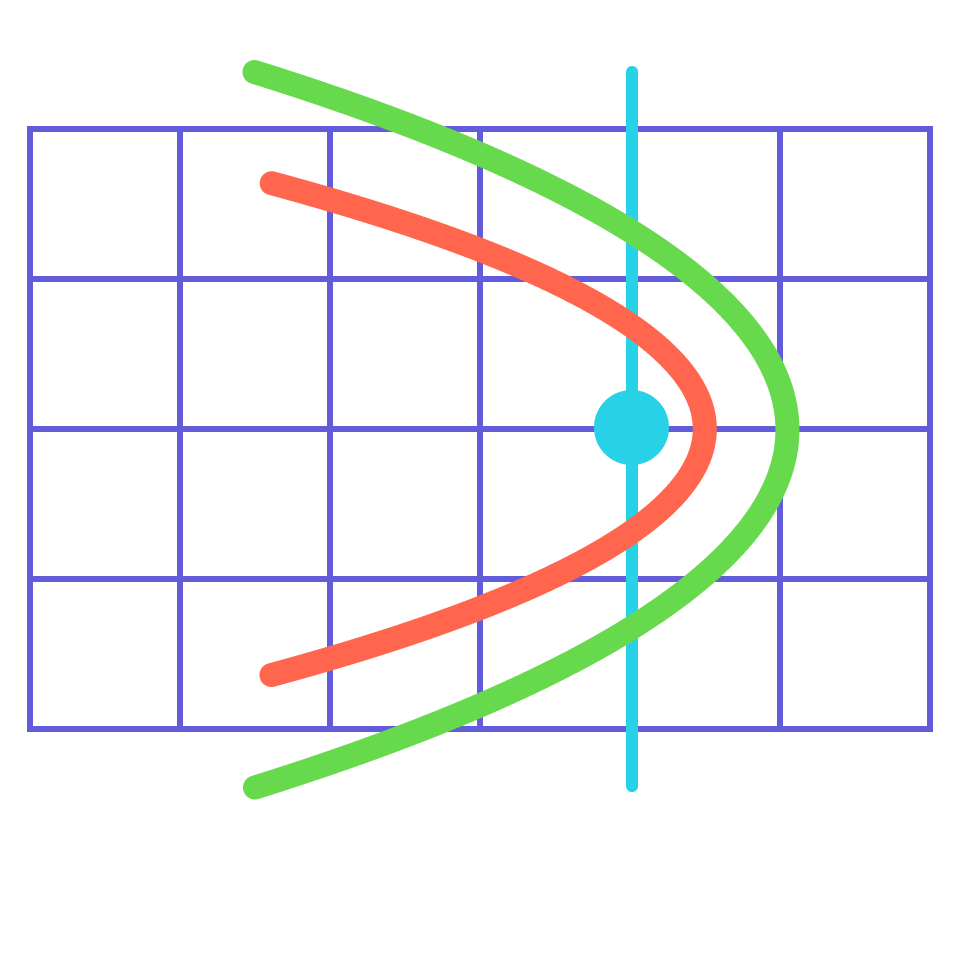# Transforming Functions

Let's continue where we left off at the end of the last quiz. Even though we don't know the equation for the function below, we know adding or subtracting the value $$Z$$ to the output will cause the entire graph to shift up or down.

$y = f(x) + Z$

The key realization here is that we don't need to know anything about the function in order to know what adding and subtracting $$Z$$ will do to the function; the transformations that you'll explore in this quiz can be applied to move any function whatsoever.

## Transforming Functions

### Introduction

# Transforming Functions

Going forward, we'll use the square root function, $$y = \sqrt{x}$$ as an example and plot $$x$$ and $$y$$ on the horizontal and vertical axes of each graph.

Which of the figures below shows the graph of the square root function, $$y = \sqrt{x}?$$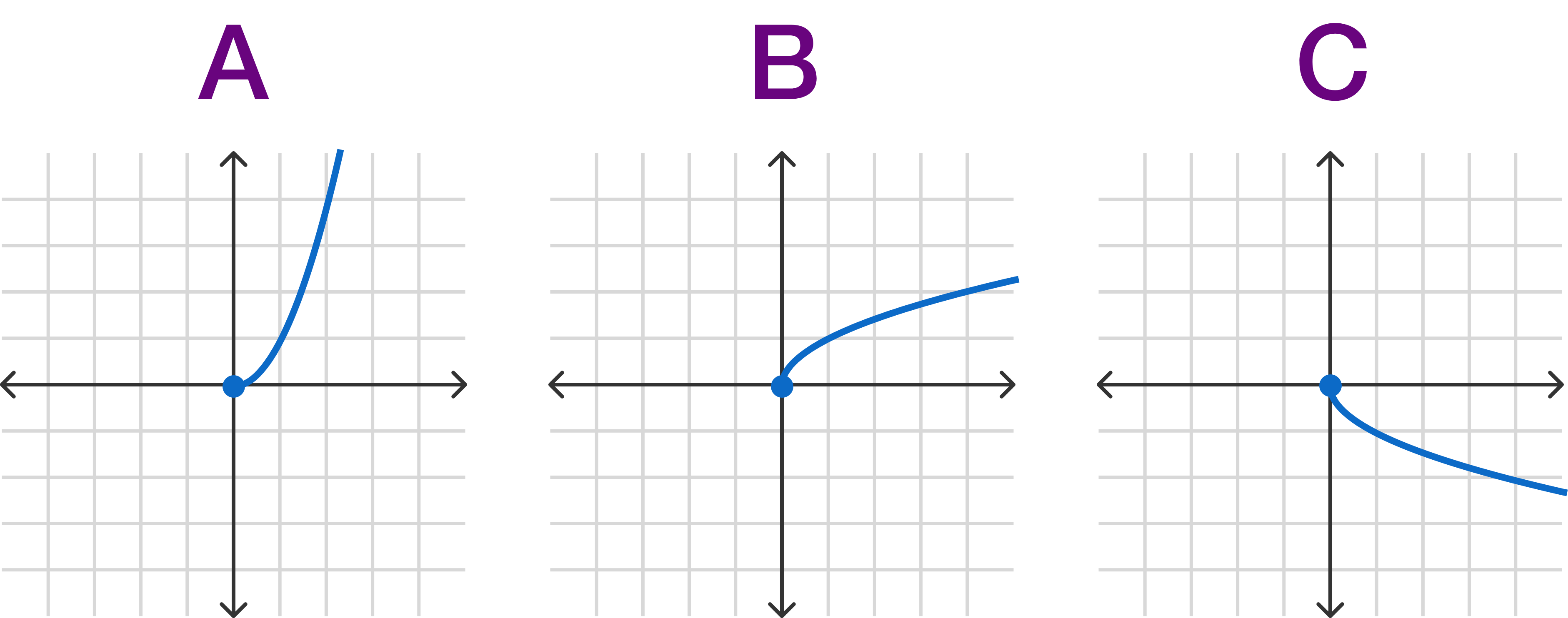## Transforming Functions

### Introduction

# Transforming Functions

As shown earlier, adding or subtracting a value to a function can cause a translation.

Which figure below is a graph of $$f(x) = \sqrt{x} + 3 ?$$## Transforming Functions

### Introduction

# Transforming FunctionsWith function transformations, it's important to distinguish changes that happen before the main function is applied (essentially, changing the input) to those that happen after the main function is applied (changing the output).

The output values are the $$y$$-coordinates, so adding or subtracting to the output will add or subtract to those directly. This is why the shifting up or down occurs.

But what about if we add to or subtract from the the input before taking the square root? That's what we'll look at next.

## Transforming Functions

### Introduction

# Transforming Functions

Which graph matches that of $$f(x)=\sqrt{x-3}?$$

Be careful! You can test values to help you out; if $$x = 3 ,$$ for instance, what is the output?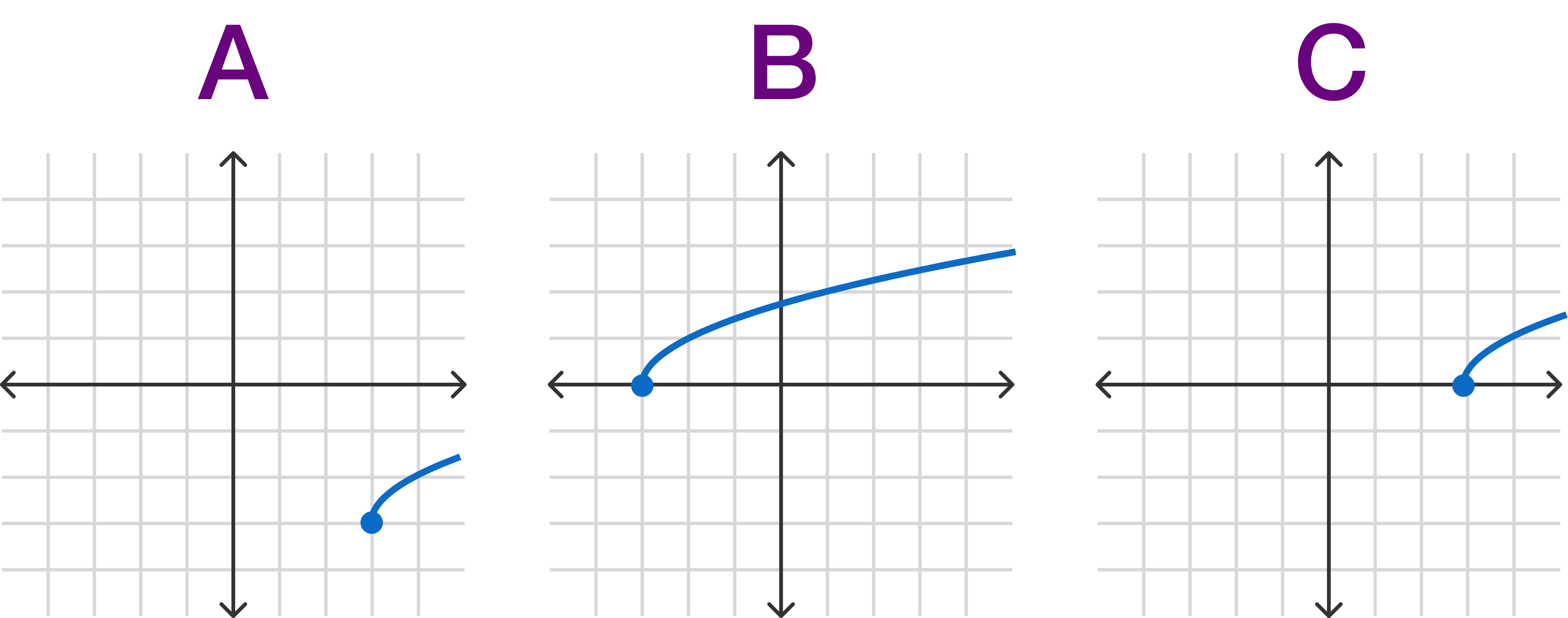## Transforming Functions

### Introduction

# Transforming Functions

If $$Q$$ is positive, what can you say in general about the transformation $$f(x+Q)$$ vs. $$f(x-Q)$$ relative to an original function $$f(x) ?$$

## Transforming Functions

### Introduction

# Transforming Functions

If you're having trouble with left and right directions being "reversed", you can think of any change to input values as happening before the graph is made. Instead of shifting the graph, this is essentially shifting the coordinate grid behind the graph. For example, if you shift the coordinate grid right by two, this is equivalent to shifting the graph of the function left by two.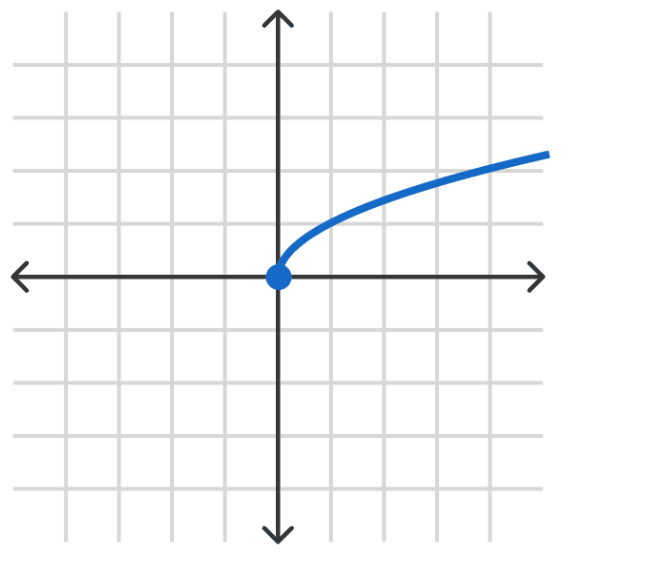## Transforming Functions

### Introduction

# Transforming Functions

Which figure below is a graph of $$f(x)=\sqrt{x+3}-2 ?$$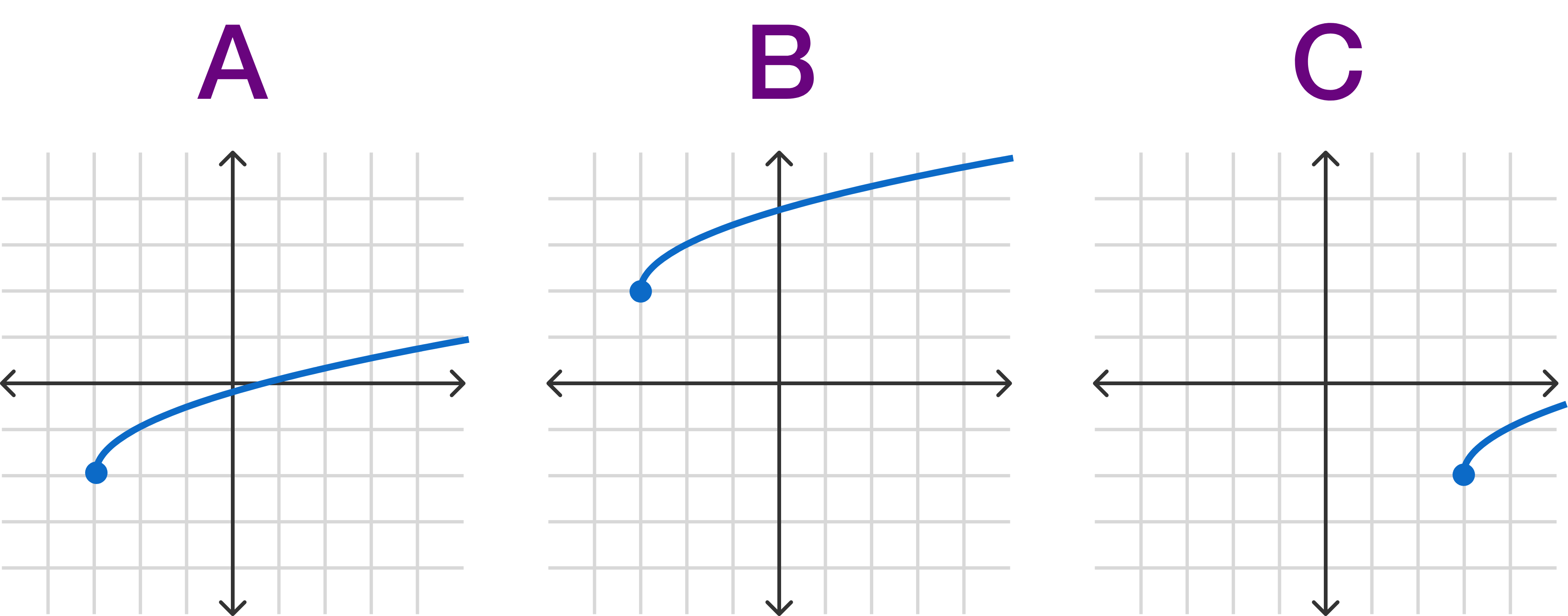## Transforming Functions

### Introduction

# Transforming Functions

To summarize, these are the function transformations we've seen so far, assuming $$Z$$ and $$Q$$ are positive:

 $$f(x) + Z$$ translate up $$f(x) - Z$$ translate down $$f(x+Q)$$ translate left $$f(x-Q)$$ translate right

The full course will expand on this list! For now though, let's move on to another critical algebraic skill: factoring.

## Transforming Functions

### Introduction

×

Problem Loading...

Note Loading...

Set Loading...